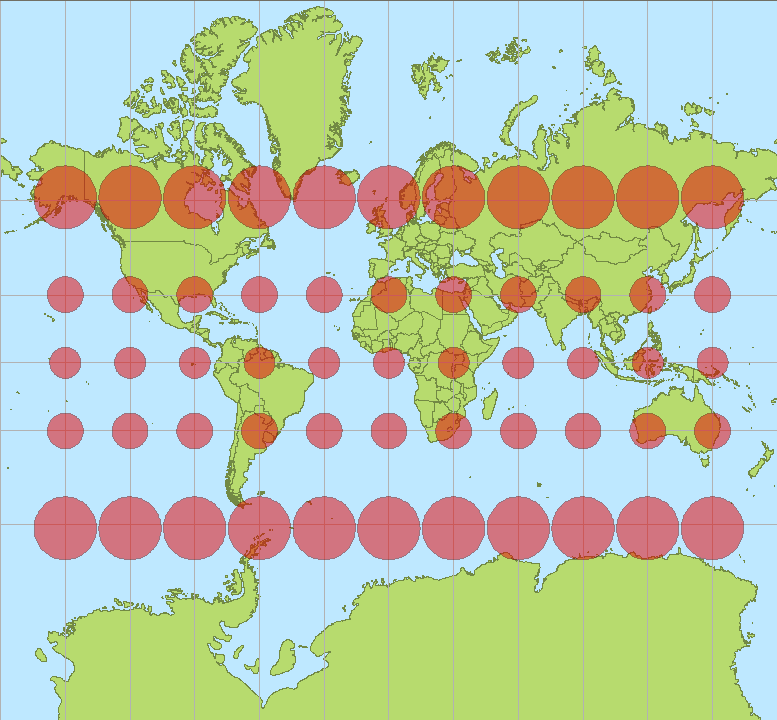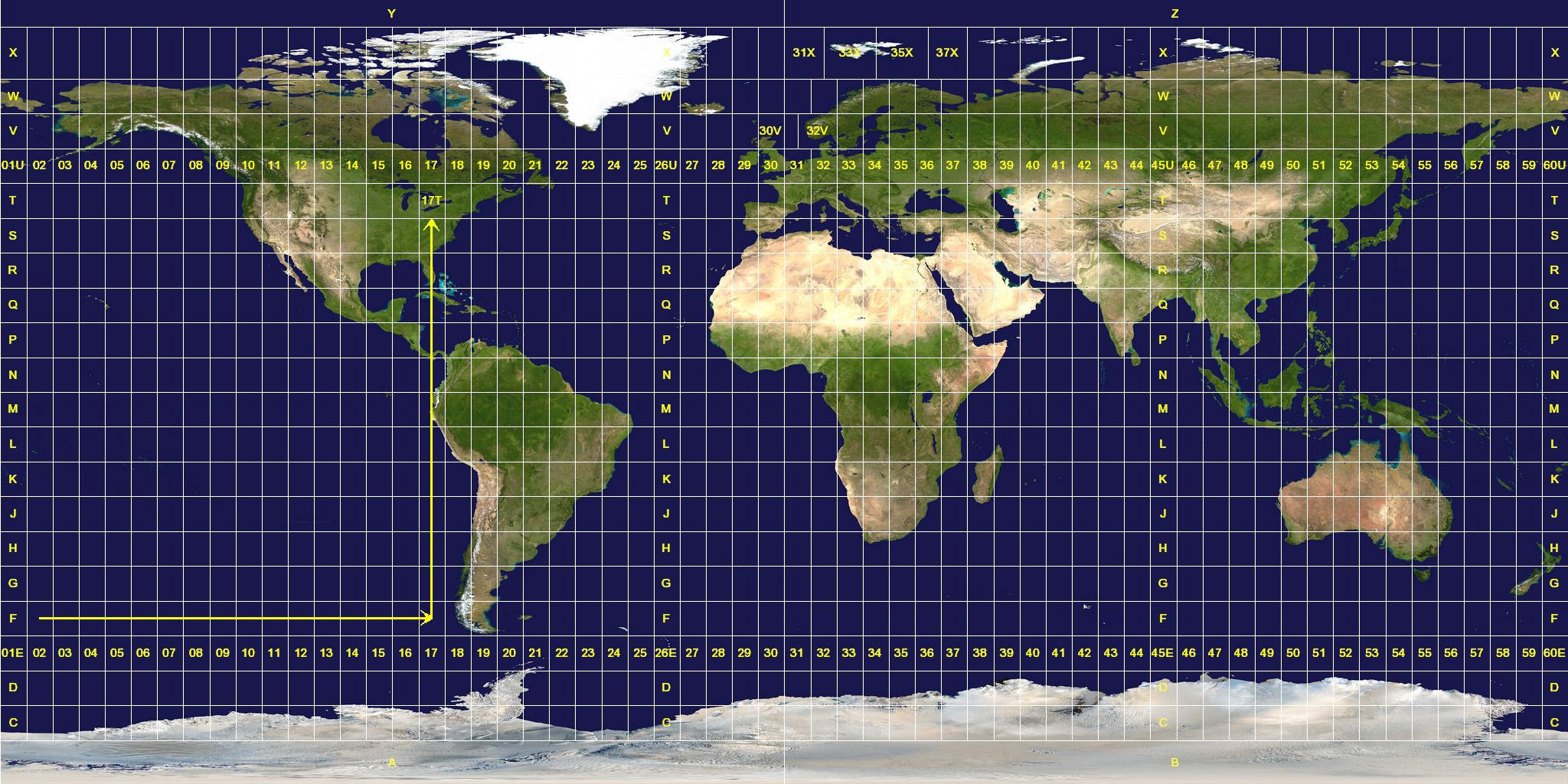An introduction to geographic coordinates

Nowadays, anybody with a smartphone can find their exact position with just one click. Any location is determined by a specific pairing of latitude and longitude in a Cartesian coordinate ellipsoid-based grid.

The actual shape of the Earth is an irregular figure called a geoid but it can be approximated (at sea level) to a mathematical ellipsoid called a datum.
The more recent and precise version of the global datum is the World Geodetic System WGS84 (established in 1984 and revised in 2004), although there are also some local datums such as the European ED50.

What is a map projection?

Although some people today still think otherwise, our planet is not flat, so any attempt to represent the Earth on a plane needs some kind of transformation, known as a map projection.In this process, there is always a compromise between the properties that get preserved and others that get deformed; any map projection therefore always has some kind of distortion. The Tissot's Indicatrix is a plot that contains circles at grid intersections and shows how they vary due to distortion.
Depending on what the map is needed for, one projection may be better than another, and there are lots of different map projections that can be classified by two main criteria:

• By the surface used:  Depending on the surface onto which the globe is projected, map projections can be cylindrical, conic or azimuthal (uses a plane).
• By the property preserved: Depending on which metric is preserved, projections can be equal-area, equidistant or conformal (angles preserved).

One of the most common projections used in the wind energy industry is the Universal Transverse Mercator (UTM).

The Universal Transverse Mercator (UTM) projection

The Mercator map projection was created by Gerardus Mercator in 1569. This projection is cylindrical and conformal, so local angles are preserved and local circles are not deformed. Distortion is constant along any parallel and constant bearings (rhumb lines) are straight lines. The map extends infinitely north and south. This is the projection used in Google Maps and, by extension, all websites feed by them, including Vortex interfaceThe Universal Transverse Mercator, however, is not a single map projection but a system that divides the Earth into sixty zones, each being a six-degree band of longitude (numbered from 01 to 60, west to east). These bands are divided into cells eight degrees high of latitude (specified by a letter from A to X, south to north, with N being the first letter north of the equator.)

A position on the Earth is given by the UTM zone number and the easting (x Cartesian coordinate) and northing (y coordinate) in that zone.

How to convert UTM to geographic coordinates (and vice versa)

UTM coordinates can be transformed to geographical coordinates using several mathematical equations. For brevity purposes, these formulae are not reproduced here but can be looked up at the Journal of Geodesy. For the sake of convenience, the form below calls a JavaScript code (thanks to Chuck Taylor) that solves these equations. Geographic coordinates are entered and displayed in decimal degrees. Negative numbers indicate West longitudes and South latitudes. UTM coordinates are entered and displayed in meters. The ellipsoid model used for computations is WGS84.

Another way to transform geographic coordinates to UTM is by using the Geospatial Data Abstraction Library (GDAL). This Open Source library can transform any coordinate system referenced to any datum. A couple of UTM coordinates (utmx, utmy) can be converted to a geographical latitude and longitude by typing:

> gdaltransform -s_srs EPSG:ZONE -t_srs EPSG:4326
utmx utmy

Where the parameter ZONE depends on the UTM zone and can be obtained from http://spatialreference.org

coordinates